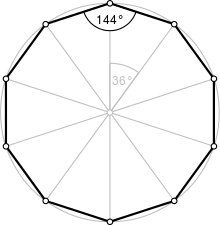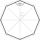# Decagon

Calculate the area and circumference of the regular decagon when its radius of a circle circumscribing is R = 1m

S =  2.9389 m2
o =  6.1803 m

### Step-by-step explanation:Did you find an error or inaccuracy? Feel free to write us. Thank you!Tips to related online calculators
Pythagorean theorem is the base for the right triangle calculator.
Do you want to convert length units?

#### You need to know the following knowledge to solve this word math problem:

We encourage you to watch this tutorial video on this math problem:

## Related math problems and questions:

• PentagonCalculate the length of side, circumference and area of a regular pentagon, which is inscribed in a circle with radius r = 6 cm.
• 30-gonAt a regular 30-gon the radius of the inscribed circle is 15cm. Find the "a" side size, circle radius "R", circumference, and content area.
• Diagonals of pentagonCalculate the diagonal length of the regular pentagon: a) inscribed in a circle of radius 12dm; b) a circumscribed circle with a radius of 12dm.Calculate the content of a regular 15-sides polygon inscribed in a circle with radius r = 4. Express the result to two decimal places.
• NonagonCalculate the area and perimeter of a regular nonagon if its radius of the inscribed circle is r = 10cm
• Hexagon ABCDEFIn the regular hexagon ABCDEF, the diagonal AE has a length 8cm. Calculate the circumference and the hexagon area.
• Regular n-gonWhich regular polygon have a radius of circumscribed circle r = 10 cm and the radius of inscribed circle p = 9.962 cm?
• Hexagon in circleCalculate the radius of a circle whose length is 10 cm greater than the circumference of a regular hexagon inscribed in this circle.
• Hexagon ACalculate area of regular hexagon inscribed in circle with radius r=9 cm.
• Right triangleCalculate the missing side b and interior angles, perimeter, and area of ​​a right triangle if a=10 cm and hypotenuse c = 16 cm.
• Arc and segmentCalculate the length of circular arc l, area of the circular arc S1 and area of circular segment S2. Radius of the circle is 11 and corresponding angle is (2)/(12) π.
• Nine-gonCalculate the perimeter of a regular nonagon (9-gon) inscribed in a circle with a radius 13 cm.
• Right triangle eq2Find the lengths of the sides and the angles in the right triangle. Given area S = 210 and perimeter o = 70.
• Pentagonal prismThe regular pentagonal prism is 10 cm high. The radius of the circle of the described base is 8 cm. Calculate the volume and surface area of the prism.
• MoonWe see Moon in the perspective angle 28'. Moon's radius is 1740 km at the time of the full moon. Calculate the mean distance of the Moon from the Earth.
• Ratio of sidesCalculate the area of a circle with the same circumference as the circumference of the rectangle inscribed with a circle with a radius of r 9 cm so that its sides are in ratio 2 to 7.
• The mastA 40 m high mast is secured in half by eight ropes of 25 m long. The ends of the ropes are equidistant from each other. Calculate this distance.